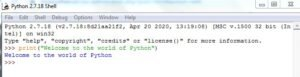# First Python Program “Hello Python”

## Introduction

Python is an interpreted language as its programs are executed by an interpreter. Thus, Python interpreter should be installed on the computer system to write and run Python programs. Python IDLE (Integrated Development and Learning Environment) provides two working modes-interactive mode (popularly known as Python shell) and script mode.

The Python IDLE tool offers an interactive and a more efficient platform to write your code in Python.

Using Interactive (Python Shell window) mode, the Python commands are directly typed at >>> (command prompt), and as soon as we press the Enter key, the interpreter displays the result(s) immediately, which is known as Displaying.

For example,

## First Python Program

Let us execute programs in different modes of programming.

### Interactive Mode Programming

Invoking the interpreter without passing a script file as a parameter brings up the following prompt −Type the following text at the Python prompt and press the Enter −

>>> print “Hello, Python!”

If you are running new version of Python, then you would need to use print statement with parenthesis as in print (“Hello, Python!”);. However in Python version 2.4.3, this produces the following result −

Hello, Python!

### Script Mode Programming

Invoking the interpreter with a script parameter begins execution of the script and continues until the script is finished. When the script is finished, the interpreter is no longer active.

Let us write a simple Python program in a script. Python files have extension .py. Type the following source code in a test.py file −

```x=5
y=3
z=x+y
z=z+3
x=y
y=5
print("The value of x is:", x)
print("The value of y is:", y)
print("The value of z is:", z)
```

We assume that you have Python interpreter set in PATH variable. Now, try to run this program as follows −

\$ python test.py

This produces the following result −

(‘The value of x is:’, 3)
(‘The value of y is:’, 5)
(‘The value of z is:’, 11)
>>>#### By cbsepython

A complete solution for the students of class 9 to 12 having subject Information Technology (402), Computer Science (083). Explore our website for all the useful content as Topic wise notes, Solved QNA, MCQs, Projects and Quiz related to the latest syllabus.